Homework Help Question & Answers

# Classify the atomic orbitals as s, p, or d according to their shape. Answer Bank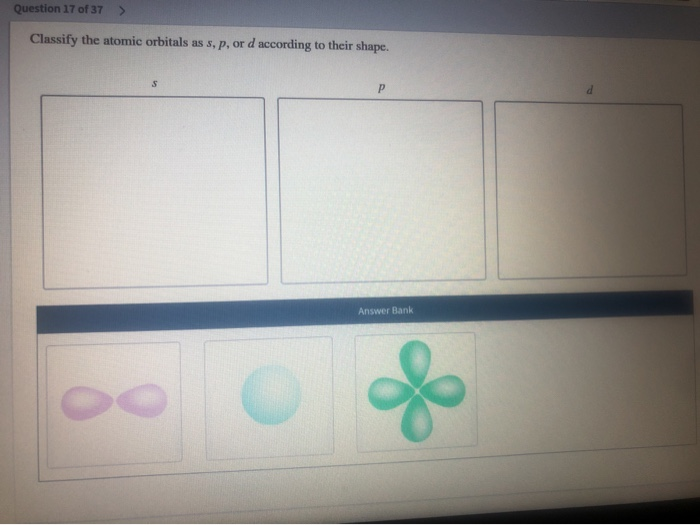Classify the atomic orbitals as s, p, or d according to their shape. Answer Bank

#### Homework Answers

Answer #2 ✔ Recommended Answer

The order of orbitals are:

s, p, d

since

s = 2 electrons ( 1 orbital, i.e. light blue sphere)

p = 6 electrons ( 6/2 = 3 orbitas, which are in x,y,z orientation, so only 2 globes per axis... ebst fit is PURPLE)

finally

d = has the most complex of all, so choose LIGHT GREEN

answered by: Affecrest
Add a comment
Answer #1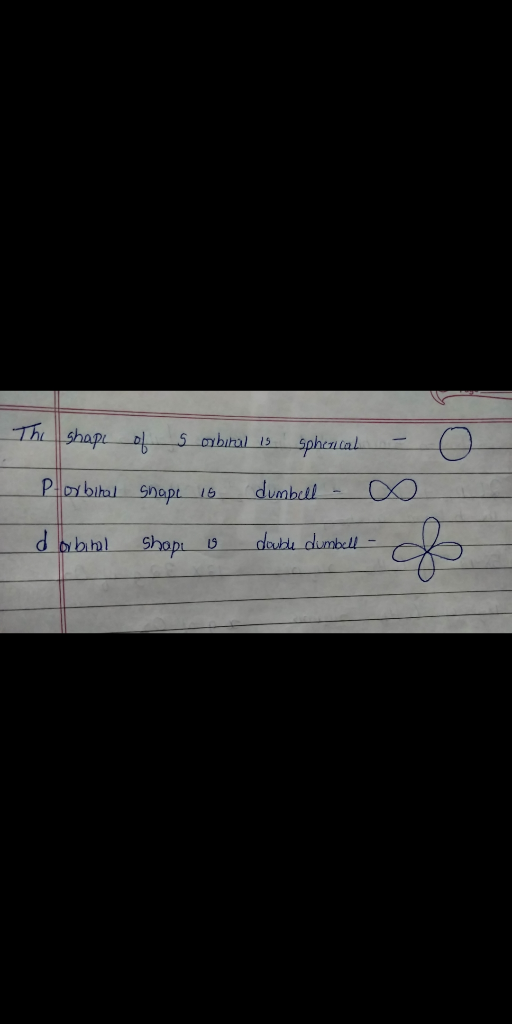Know the answer?
Your Answer:

#### Post as a guest

Your Name:

What's your source?

#### Earn Coin

Coins can be redeemed for fabulous gifts.

Not the answer you're looking for? Ask your own homework help question. Our experts will answer your question WITHIN MINUTES for Free.
Similar Homework Help Questions
• ### (e) a- use of s and p atomic orbitals b- use of s, p, and d...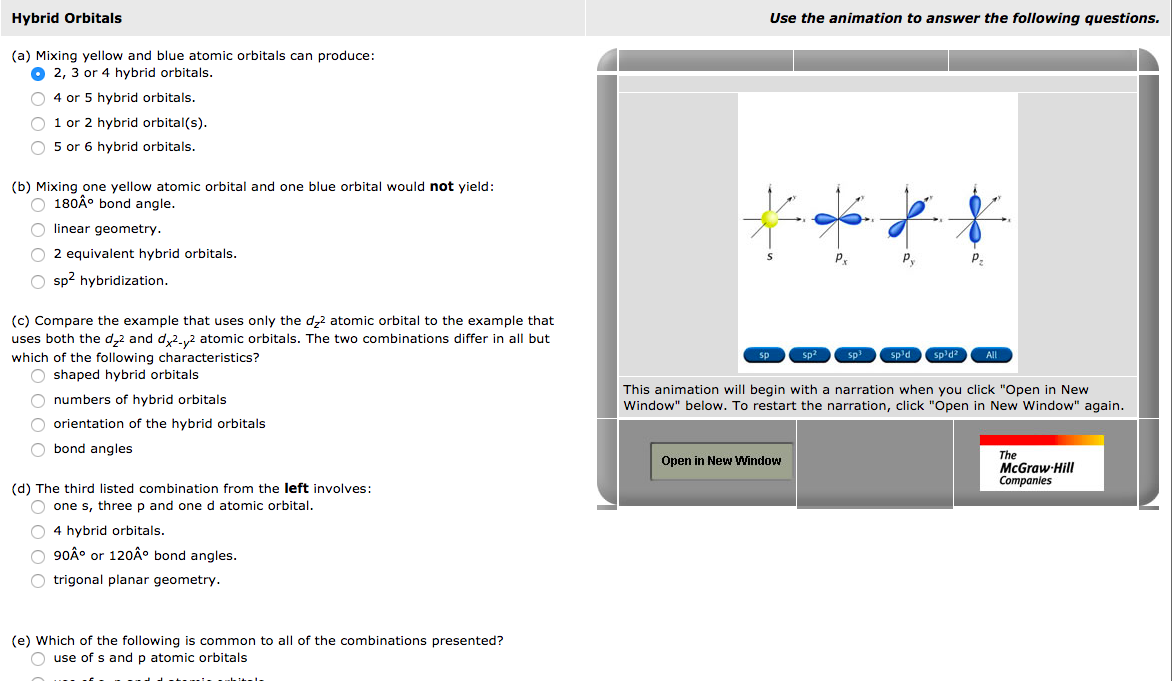(e) a- use of s and p atomic orbitals b- use of s, p, and d atomic orbitals c-use of only s orbitals d-generation of more than four hybrid orbitals Hybrid Orbitals Use the animation to answer the following questions. (a) Mixing yellow and blue atomic orbitals can produce: 2, 3 or 4 hybrid orbitals. O 4 or 5 hybrid orbitals. 1 or 2 hybrid orbital(s). O 5 or 6 hybrid orbitals. (b) Mixing one yellow atomic orbital and one...

• ### a. b. Answer the questions below for 1,3,5,7-decatetraene. How many p atomic orbitals are in the...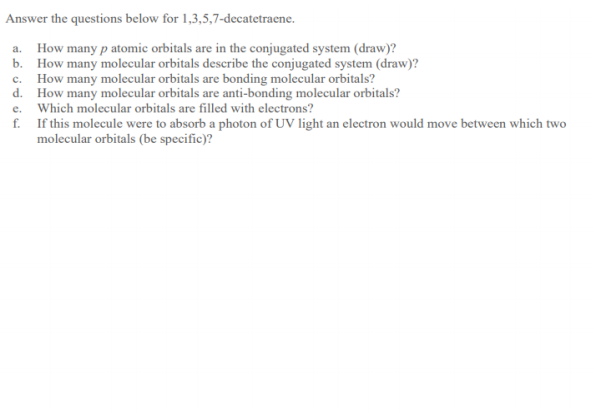a. b. Answer the questions below for 1,3,5,7-decatetraene. How many p atomic orbitals are in the conjugated system (draw)? How many molecular orbitals describe the conjugated system (draw)? How many molecular orbitals are bonding molecular orbitals? d. How many molecular orbitals are anti-bonding molecular orbitals? e. Which molecular orbitals are filled with electrons? f. If this molecule were to absorb a photon of UV light an electron would move between which two molecular orbitals (be specific)?

• ### 9. Answer the questions below for 1,3,5,7-decatetraene. a. How many p atomic orbitals are in the...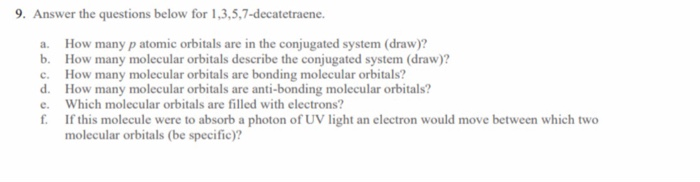9. Answer the questions below for 1,3,5,7-decatetraene. a. How many p atomic orbitals are in the conjugated system (draw)? b. How many molecular orbitals describe the conjugated system (draw)? c. How many molecular orbitals are bonding molecular orbitals? d. How many molecular orbitals are anti-bonding molecular orbitals? e. Which molecular orbitals are filled with electrons? f. If this molecule were to absorb a photon of UV light an electron would move between which two molecular orbitals (be specific)?

• ### Determine the point groups of the p and d atomic orbitals, including signs/phases on the orbital...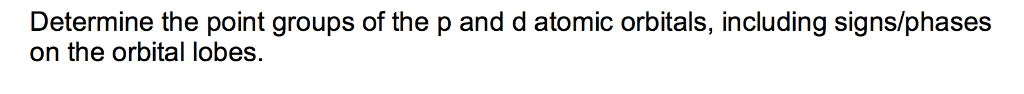Determine the point groups of the p and d atomic orbitals, including signs/phases on the orbital lobes.

• ### Answer the questions below for 1,3,5,7-decatetraene. c. How many p atomic orbitals are in the conjugated...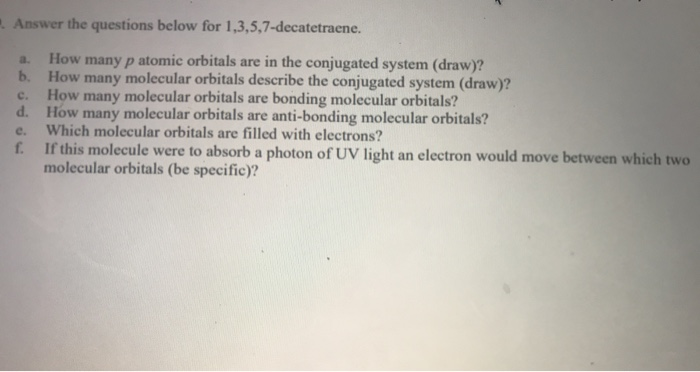Answer the questions below for 1,3,5,7-decatetraene. c. How many p atomic orbitals are in the conjugated system (draw)? b. How many molecular orbitals describe the conjugated system (draw)? How many molecular orbitals are bonding molecular orbitals? d. How many molecular orbitals are anti-bonding molecular orbitals? Which molecular orbitals are filled with electrons? f. If this molecule were to absorb a photon of UV light an electron would move between which two molecular orbitals (be specific)? e.

• ### Classify these orbital descriptions by type. Atomic orbital Hybrid orbital Molecular orbital p d f P...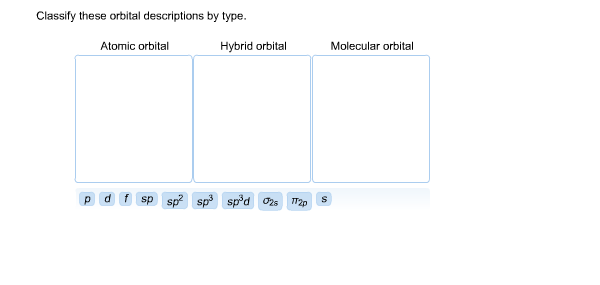Classify these orbital descriptions by type. Atomic orbital Hybrid orbital Molecular orbital p d f P sp^2 sp^3 sp^3d sigma2s pi2p S

• ### 5. Molecular orbitals are formed by the overlap of atomic orbitals. Thus, n atomic orbitals can combine (overlap) t...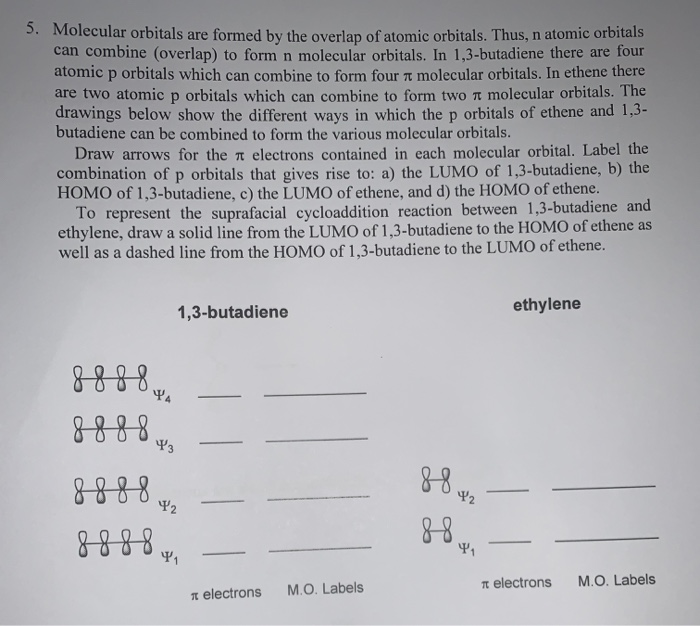5. Molecular orbitals are formed by the overlap of atomic orbitals. Thus, n atomic orbitals can combine (overlap) to form n molecular orbitals. In 1,3-butadiene there are four atomic p orbitals which can combine to form four 7 molecular orbitals. In ethene there are two atomic p orbitals which can combine to form two y molecular orbitals. The drawings below show the different ways in which the p orbitals of ethene and 1,3- butadiene can be combined to form the...

• ### The T bond in ethylene, CH 2CH 2, results from the overlap of s atomic orbitals...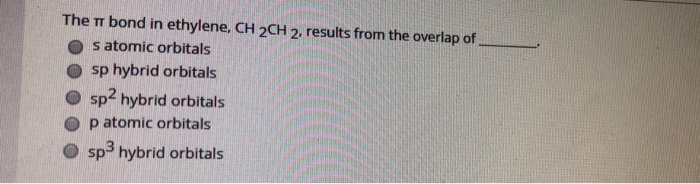The T bond in ethylene, CH 2CH 2, results from the overlap of s atomic orbitals sp hybrid orbitals sp2 hybrid orbitals p atomic orbitals sp hybrid orbitals

• ### Figure 6.11 shows the atomic s and p orbitals in a chain of atoms and how...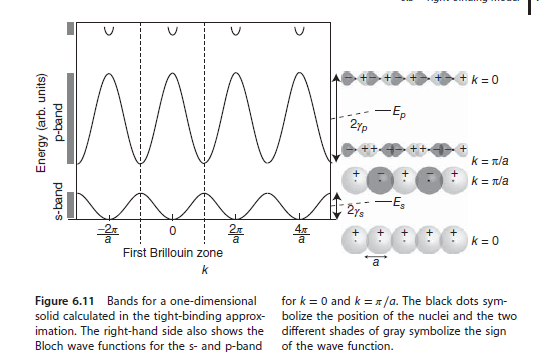Figure 6.11 shows the atomic s and p orbitals in a chain of atoms and how these are combined to form the bonding and antibonding states. For the s band, the bonding state is formed with the atomic wave functions on all sites combined in phase, corresponding to a wave vector k = 0, and the antibonding state is associated with a sign change of the wave function on every other site, corresponding to a wave vector at the Brillouin...

• ### Sketch the bonding and antibondong pi orbitals from two atomic p orbitals and discuss their relative...

Sketch the bonding and antibondong pi orbitals from two atomic p orbitals and discuss their relative energies and overlap integral

Free Homework App

Scan Your Homework
to Get Instant Free Answers
Need Online Homework Help?

Get Answers For Free
Most questions answered within 3 hours.